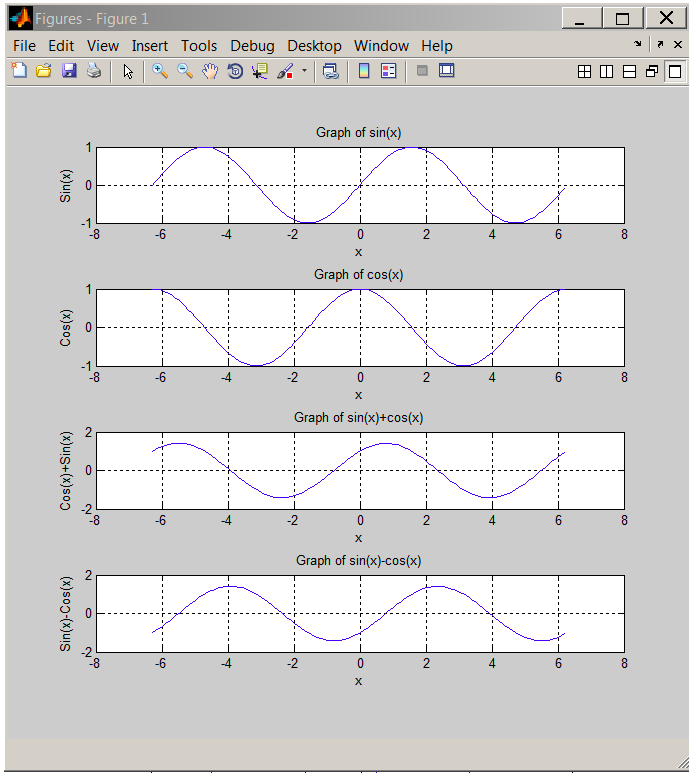6.1 Plotting in MATLABA picture is worth a thousand words, particularly visual representation of data in engineering is very useful. MATLAB has powerful graphics tools and there is a very helpful section devoted to graphics in MATLAB Help: Graphics. Students are encouraged to study that section; what follows is a brief summary of the main plotting features.

Two-Dimensional Plots

The

plot


Statement

Probably the most common method for creating a plot is by issuing plot(x, y) statement where function y is plotted against x.

Type in the following statement at the MATLAB prompt:

x=[-pi:.1:pi]; y=sin(x); plot(x,y);


After we executed the statement above, a plot named Figure1 is generated:$$\PageIndex{1}$$ Graph of sin(x)

Having variables assigned in the Workspace, x and y=sin(x) in our case, we can also select x and y, and right click on the selected variables. This opens a menu from which we choose plot(x,y). See the figure below.$$\PageIndex{2}$$ Creating a plot from Workspace.

Annotating Plots

Graphs without labels are incomplete and labeling elements such as plot title, labels for x and y axes, and legend should be included. Using up arrow, recall the statement above and add the annotation commands as shown below.

ParseError: invalid XmlNode (click for details)
Callstack:
at (Under_Construction/Book:_A_Brief_Introduction_to_Engineering_Computation_with_MATLAB_(Beyenir)/06:_Graphics), /content/body/div/div/div/pre, line 3, column 41


Run the file and compare your result with the first one.$$\PageIndex{3}$$ Graph of sin(x) with Labels.

aside:

Type in the following at the MATLAB prompt and learn additional commands to annotate plots:

help gtext
help legend
help zlabel


Superimposed Plots

If you want to merge data from two graphs, rather than create a new graph from scratch, you can superimpose the two using a simple trick:

ParseError: invalid XmlNode (click for details)
Callstack:
at (Under_Construction/Book:_A_Brief_Introduction_to_Engineering_Computation_with_MATLAB_(Beyenir)/06:_Graphics), /content/body/div/div/div/pre, line 10, column 8$$\PageIndex{4}$$ Graph of sin(x) and cos(x) in the same plot with labels and legend.

Multiple Plots in a Figure

Multiple plots in a single figure can be generated with subplot in the Command Window. However, this time we will use the built-in Plot Tools. Before we initialize that tool set, let us create the necessary variables using the following script:

% This script generates sin(x) and cos(x) variables
clc                 %Clears command window
clear all           %Clears the variable space
close all           %Closes all figures
X1=[-2*pi:.1:2*pi];  %Creates a row vector from -2*pi to 2*pi with .1 increments
Y1=sin(X1);          %Calculates sine value for each x
Y2=cos(X1);          %Calculates cosine value for each x
Y3=Y1+Y2;            %Calculates sin(x)+cos(x)
Y4=Y1-Y2;            %Calculates sin(x)-cos(x)


Note that the above script clears the command window and variable workspace. It also closes any open Figures. After running the script, we will have X1, Y1, Y2, Y3 and Y4 loaded in the workspace. Next, select File > New > Figure, a new Figure window will open. Click "Show Plot Tools and Dock Figure" on the tool bar.$$\PageIndex{5}$$ Plot Tools

Under New Subplots > 2D Axes, select four vertical boxes that will create four subplots in one figure. Also notice, the five variables we created earlier are listed under Variables.$$\PageIndex{6}$$ Creating four sub plots.

After the subplots have been created, select the first supblot and click on "Add Data". In the dialog box, set X Data Source to X1 and Y Data Source to Y1. Repeat this step for the remaining subplots paying attention to Y Data Source (Y2, Y3 and Y4 need to be selected in the subsequent steps while X1 is always the X Data Source).$$\PageIndex{7}$$ Adding data to axes.

Next, select the first item in "Plot Browser" and activate the "Property Editor". Fill out the fields as shown in the figure below. Repeat this step for all subplots.$$\PageIndex{8}$$ Using "Property Editor".

Save the figure as sinxcosx.fig in the current directory.$$\PageIndex{9}$$ The four subplots generated with "Plot Tools".$$\PageIndex{10}$$ The four subplots in a single figure.

Three-Dimensional Plots

3D plots can be generated from the Command Window as well as by GUI alternatives. This time, we will go back to the Command Window.

The

plot3


Statement

With the X1,Y1,Y2 and Y2 variables still in the workspace, type in plot3(X1,Y1,Y2) at the MATLAB prompt. A figure will be generated, click "Show Plot Tools and Dock Figure".$$\PageIndex{11}$$ A raw 3D figure is generated with plot3.

Use the property editor to make the following changes.$$\PageIndex{12}$$ 3D Property Editor.

The final result should look like this:$$\PageIndex{13}$$ 3D graph of x, sin(x), cos(x)

Use help or doc commands to learn more about 3D plots, for example, image(x), surf(x) and mesh(x).

Quiver or Velocity Plots

To plot vectors, it is useful to draw arrows so that the direction of the arrow points the direction of the vector and the length of the arrow is vector’s magnitude. However the standard plot function is not suitable for this purpose. Fortunately, MATLAB has quiverfunction appropriately named to plot arrows. quiver(x,y,u,v) plots vectors as arrows at the coordinates (x,y) with components (u,v). The matrices x, y, u, and v must all be the same size and contain corresponding position and velocity components.

Calculate the magnitude of forces OA, OB and the resultant R of OA and OB shown below. Plot all three forces on x-y Cartesian coordinate system1.$$\PageIndex{14}$$ Quiver Plot.

ParseError: invalid XmlNode (click for details)
Callstack:
at (Under_Construction/Book:_A_Brief_Introduction_to_Engineering_Computation_with_MATLAB_(Beyenir)/06:_Graphics), /content/body/div/section/div/div/div/section/div/div/div/pre, line 17, column 8$$\PageIndex{15}$$ Output of quiver function.

Write an interactive script to calculate the resultant R of forces F1, F2 and F3 shown below and plot all four forces on x-y Cartesian coordinate system2.$$\PageIndex{16}$$ An example for quiver3 plot.

ParseError: invalid XmlNode (click for details)
Callstack:
at (Under_Construction/Book:_A_Brief_Introduction_to_Engineering_Computation_with_MATLAB_(Beyenir)/06:_Graphics), /content/body/div/section/div/div/div/section/div/div/div/pre, line 5, column 7$$\PageIndex{17}$$ Output of quiver3 function.

Summary of Key Points

1. plot(x, y) and plot3(X1,Y1,Y2) statements create 2- and 3-D graphs respectively,
2. Plots at minimum should contain the following elements: title, xlabel, ylabel and legend,
3. Annotated plots can be easily generated with GUI Plot Tools,
4. quiver and quiver3 plots are useful for making vector diagrams.

Footnotes

• 1 Applied Engineering Mechanics by A. Jensen, H. Chenoweth McGraw-Hill Ryerson Limited © 1972, (p. 15)
• 2 Applied Engineering Mechanics by A. Jensen, H. Chenoweth McGraw-Hill Ryerson Limited © 1972, (p. 15)